# NCERT Solutions class 10 maths ch-1 Real Numbers

## EX- 1.1

Question 1.
Use Euclid’s Division Algorithm to find the HCF of:
(i) 135 and 225
(ii) 196 and 38220
(iii) 867 and 255
Solution:
(i)  135 and 225
225 > 135,
225 = 135 × 1 + 90

Also,
135 = 90 × 1 + 45
90 = 2 × 45 + 0
Therefore, the HCF of 135 and 225 is 45.

(ii). 196 and 38220

In this given question, 38220>196, therefore the by applying Euclid’s division algorithm and taking 38220 as divisor, we get,

38220 = 196 × 195 + 0

We have already got the remainder as 0 here. Therefore, HCF(196, 38220) = 196.

Hence, the HCF of 196 and 38220 is 196.

(iii). 867 and 225

As we know, 867 is greater than 225. Let us apply now Euclid’s division algorithm on 867, to get,

867 = 225 × 3 + 102

Remainder 102 ≠ 0, therefore taking 225 as divisor and applying the division lemma method, we get,

225 = 102 × 2 + 51

Again, 51 ≠ 0. Now 102 is the new divisor, so repeating the same step we get,

102 = 51 × 2 + 0

The remainder is now zero, so our procedure stops here. Since, in the last step, the divisor is 51, therefore, HCF (867,225) = HCF(225,102) = HCF(102,51) = 51.

Hence, the HCF of 867 and 225 is 51.

Question 2.
Show that any positive odd integer is of the form 6q + 1, or 6q + 3, or 6q + 5, where q is some integer.
Solution
By Euclid’s algorithm,

a = 6q + for some integer q ≥ 0, and r = 0, 1, 2, 3, 4, 5 because 0 ≤ r < 6.

Therefore, a = 6q or 6q + 1 or 6q + 2 or 6q + 3 or 6q + 4 or 6q + 5

Also, 6q + 1 = 2 x 3q + 1=2k+1, where  is a positive integer

6q + 3 = (6q + 2) + 1 = 2 (3q + 1) + 1 =, where  is an integer

6q + 5 = (6q + 4) + 1 = 2 (3q + 2) + 1 =, where  is an integer

Clearly, 6q + 1, 6q + 3, 6q + 5 are of the form 2k + 1, where k is an integer.

Therefore, 6q + 1, 6q + 3, 6q + 5 are not exactly divisible by 2. Hence, these expressions of numbers are odd numbers.

And therefore, any odd integer can be expressed in the form 6q + 1, or 6q + 3,

Question 3
An army contingent of 616 members is to march behind an army band of 32 members in a parade. The two groups are to march in the same number of columns. What is the maximum number of columns in which they can march?
Ans. We have to find the HCF (616, 32) to find the maximum number of columns in which they can march.

To find the HCF, we can use Euclid’s algorithm.

The HCF (616, 32) is 8.

Therefore, they can march in 8 columns each.

Question 4
Use Euclid’s division lemma to show that the square of any positive integer is either of form 3m or 3m + 1 for some integer m. [Hint: Let x be any positive integer then it is of the form 3q, 3q + 1 or 3q 2. Now square each of these and show that they can be rewritten in the form 3m or 3m + 1.]
Ans. Let a be any positive integer and b = 3.

Then a = 3q + r for some integer q ≥ 0

And r = 0, 1, 2 because 0 ≤ r < 3

Therefore, a = 3q or 3q + 1 or 3q + 2

Or,Hence, it can be said that the square of any positive integer is either of the form 3m or 3m + 1.

Question 5
Use Euclid’s Division Lemma to show that the cube of any positive integer is either of the form 9m, 9m + 1 or 9m + 8.
Solution:

Let x be any positive integer and y = 3.

By Euclid’s division algorithm, then,

x = 3q+r, where q≥0 and r = 0, 1, 2, as r ≥ 0 and r < 3.

Therefore, putting the value of r, we get,

x = 3q

or

x = 3q + 1

or

x = 3q + 2

Now, by taking the cube of all the three above expressions, we get,

Case (i): When r = 0, then,

x2= (3q)3 = 27q3= 9(3q3)= 9m; where m = 3q3

Case (ii): When r = 1, then,

x3 = (3q+1)3 = (3q)+13+3×3q×1(3q+1) = 27q3+1+27q2+9q

Taking 9 as common factor, we get,

x= 9(3q3+3q2+q)+1

Putting = m, we get,

Putting (3q3+3q2+q) = m, we get ,

x3 = 9m+1

Case (iii): When r = 2, then,

x3 = (3q+2)3= (3q)3+23+3×3q×2(3q+2) = 27q3+54q2+36q+8

Taking 9 as common factor, we get,

x3=9(3q3+6q2+4q)+8

Putting (3q3+6q2+4q) = m, we get ,

x3 = 9m+8

Therefore, from all the three cases explained above, it is proved that the cube of any positive integer is of the form 9m, 9m + 1 or 9m + 8.

## Ex 1.2

Question 1.

Express each number as a product of its prime factors:
(i) 140
(ii) 156
(iii) 3825
(iv) 5005
(v) 7429
Solution:

(i) 140

By Taking the LCM of 140, we will get the product of its prime factor.

Therefore, 140 = 2 × 2 × 5 × 7 × 1 = 22×5×7

(ii) 156

By Taking the LCM of 156, we will get the product of its prime factor.

Hence, 156 = 2 × 2 × 13 × 3 × 1 = 22× 13 × 3

(iii) 3825

By Taking the LCM of 3825, we will get the product of its prime factor.

Hence, 3825 = 3 × 3 × 5 × 5 × 17 × 1 = 32×52×17

(iv) 5005

By Taking the LCM of 5005, we will get the product of its prime factor.

Hence, 5005 = 5 × 7 × 11 × 13 × 1 = 5 × 7 × 11 × 13

(v) 7429

By Taking the LCM of 7429, we will get the product of its prime factor.

Hence, 7429 = 17 × 19 × 23 × 1 = 17 × 19 × 23

Question 2.
Find the LCM and HCF of the following pairs of integers and verify that LCM x HCF = Product of the two numbers:
(i) 26 and 91
(ii) 510 and 92
(iii) 336 and 54
Solution:

(i) 26 and 91

Expressing 26 and 91 as product of its prime factors, we get,

26 = 2 × 13 × 1

91 = 7 × 13 × 1

Therefore, LCM (26, 91) = 2 × 7 × 13 × 1 = 182

And HCF (26, 91) = 13

Verification

Now, product of 26 and 91 = 26 × 91 = 2366

And Product of LCM and HCF = 182 × 13 = 2366

Hence, LCM × HCF = product of the 26 and 91.

(ii) 510 and 92

Expressing 510 and 92 as product of its prime factors, we get,

510 = 2 × 3 × 17 × 5 × 1

92 = 2 × 2 × 23 × 1

Therefore, LCM(510, 92) = 2 × 2 × 3 × 5 × 17 × 23 = 23460

And HCF (510, 92) = 2

Verification

Now, product of 510 and 92 = 510 × 92 = 46920

And Product of LCM and HCF = 23460 × 2 = 46920

Hence, LCM × HCF = product of the 510 and 92.

(iii) 336 and 54

Expressing 336 and 54 as product of its prime factors, we get,

336 = 2 × 2 × 2 × 2 × 7 × 3 × 1

54 = 2 × 3 × 3 × 3 × 1

Therefore, LCM(336, 54) = = 3024

And HCF(336, 54) = 2×3 = 6

Verification

Now, product of 336 and 54 = 336 × 54 = 18,144

And Product of LCM and HCF = 3024 × 6 = 18,144

Hence, LCM × HCF = product of the 336 and 54.

3. Find the LCM and HCF of the following integers by applying the prime factorisation method.

(i) 12, 15 and 21

(ii) 17, 23 and 29

(iii) 8, 9 and 25

Solutions:

(i) 12, 15 and 21

Writing the product of prime factors for all the three numbers, we get,

12=2×2×3

15=5×3

21=7×3

Therefore,

HCF(12,15,21) = 3

LCM(12,15,21) = 2 × 2 × 3 × 5 × 7 = 420

(ii) 17, 23 and 29

Writing the product of prime factors for all the three numbers, we get,

17=17×1

23=23×1

29=29×1

Therefore,

HCF(17,23,29) = 1

LCM(17,23,29) = 17 × 23 × 29 = 11339

(iii) 8, 9 and 25

Writing the product of prime factors for all the three numbers, we get,

8=2×2×2×1

9=3×3×1

25=5×5×1

Therefore,

HCF(8,9,25)=1

LCM(8,9,25) = 2×2×2×3×3×5×5 = 1800

4. Given that HCF (306, 657) = 9, find LCM (306, 657).

Solution: As we know that,

HCF×LCM=Product of the two given numbers

Therefore,

9 × LCM = 306 × 657

LCM = (306×657)/9 = 22338

Hence, LCM(306,657) = 22338

5. Check whether 6n can end with the digit 0 for any natural number n.

Solution: If the number 6n ends with the digit zero (0), then it should be divisible by 5, as we know any number with unit place as 0 or 5 is divisible by 5.

Prime factorization of 6n = (2×3)n

Therefore, the prime factorization of 6n doesn’t contain prime number 5.

Hence, it is clear that for any natural number n, 6n is not divisible by 5 and thus it proves that 6n cannot end with the digit 0 for any natural number n.

6. Explain why 7 × 11 × 13 + 13 and 7 × 6 × 5 × 4 × 3 × 2 × 1 + 5 are composite numbers.

Solution: By the definition of composite number, we know, if a number is composite, then it means it has factors other than 1 and itself. Therefore, for the given expression;

7 × 11 × 13 + 13

Taking 13 as common factor, we get,

=13(7×11×1+1) = 13(77+1) = 13×78 = 13×3×2×13

Hence, 7 × 11 × 13 + 13 is a composite number.

Now let’s take the other number,

7 × 6 × 5 × 4 × 3 × 2 × 1 + 5

Taking 5 as a common factor, we get,

=5(7×6×4×3×2×1+1) = 5(1008+1) = 5×1009

Hence, 7 × 6 × 5 × 4 × 3 × 2 × 1 + 5 is a composite number.

7. There is a circular path around a sports field. Sonia takes 18 minutes to drive one round of the field, while Ravi takes 12 minutes for the same. Suppose they both start at the same point and at the same time, and go in the same direction. After how many minutes will they meet again at the starting point?

Solution: Since, Both Sonia and Ravi move in the same direction and at the same time, the method to find the time when they will be meeting again at the starting point is LCM of 18 and 12.

Therefore, LCM(18,12) = 2×3×3×2×1=36

Hence, Sonia and Ravi will meet again at the starting point after 36 minutes.

## Ex 1.3

1. Prove that √is irrational.

Solutions: Let us assume, that 5 is rational number.

i.e. 5 = x/y (where, x and y are co-primes)

y5= x

Squaring both the sides, we get,

(y5)2 = x2

⇒5y2 = x2……………………………….. (1)

Thus, x2 is divisible by 5, so x is also divisible by 5.

Let us say, x = 5k, for some value of k and substituting the value of x in equation (1), we get,

5y2 = (5k)2

⇒y2 = 5k2

is divisible by 5 it means y is divisible by 5.

Therefore, x and y are co-primes. Since, our assumption about  is rational is incorrect.

Hence, 5 is irrational number.

2. Prove that 3 + 2√5 + is irrational.

Solutions: Let us assume 3 + 25 is rational.

Then we can find co-prime x and y (y ≠ 0) such that 3 + 2√5 = x/y

Rearranging, we get,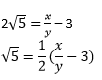Since, x and y are integers, thus,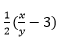is a rational number.

Therefore, 5 is also a rational number. But this contradicts the fact that 5 is irrational.

So, we conclude that 3 + 25 is irrational.

3. Prove that the following are irrationals:

(i) 1/√2

(ii) 7√5

(iii) 6 + 2

Solutions:

(i) 1/2

Let us assume 1/√2 is rational.

Then we can find co-prime x and y (y ≠ 0) such that 1/√2 = x/y

Rearranging, we get,

√2 = y/x

Since, x and y are integers, thus, √2 is a rational number, which contradicts the fact that √2 is irrational.

Hence, we can conclude that 1/√2 is irrational.

(ii) 75

Let us assume 7√5 is a rational number.

Then we can find co-prime a and b (b ≠ 0) such that 7√5 = x/y

Rearranging, we get,

√5 = x/7y

Since, x and y are integers, thus, √5 is a rational number, which contradicts the fact that √5 is irrational.

Hence, we can conclude that 7√5 is irrational.

(iii) 6 +2

Let us assume 6 +√2 is a rational number.

Then we can find co-primes x and y (y ≠ 0) such that 6 +√2 = x/y⋅

Rearranging, we get,

√2 = (x/y) – 6

Since, x and y are integers, thus (x/y) – 6 is a rational number and therefore, √2 is rational. This contradicts the fact that √2 is an irrational number.

Hence, we can conclude that 6 +√2 is irrational.

## Ex 1.4

1. Without actually performing the long division, state whether the following rational numbers will have a terminating decimal expansion or a non-terminating repeating decimal expansion:

(i) 13/3125 (ii) 17/8 (iii) 64/455 (iv) 15/1600 (v) 29/343 (vi) 23/(2352) (vii) 129/(225775) (viii) 6/15 (ix) 35/50 (x) 77/210

Solutions:

Note: If the denominator has only factors of 2 and 5 or in the form of 2m ×5n then it has terminating decimal expansion.

If the denominator has factors other than 2 and 5 then it has a non-terminating decimal expansion.

(i) 13/3125

Factorizing the denominator, we get,

3125 = 5 × 5 × 5 = 55

Since, the denominator has only 5 as its factor, 13/3125 has a terminating decimal expansion.

(ii) 17/8

Factorizing the denominator, we get,

8 = 2×2×2 = 23

Since, the denominator has only 2 as its factor, 17/8 has a terminating decimal expansion.

(iii) 64/455

Factorizing the denominator, we get,

455 = 5×7×13

Since, the denominator is not in the form of 2m × 5n, thus 64/455 has a non-terminating decimal expansion.

(iv) 15/ 1600

Factorizing the denominator, we get,

1600 = 2652

Since, the denominator is in the form of 2m × 5n, thus 15/1600 has a terminating decimal expansion.

(v) 29/343

Factorizing the denominator, we get,

343 = 7×7×7 = 73 Since, the denominator is not in the form of 2m × 5n thus 29/343 has a non-terminating decimal expansion.

(vi)23/(2352)

Clearly, the denominator is in the form of 2m × 5n.

Hence, 23/ (2352) has a terminating decimal expansion.

(vii) 129/(225775)

As you can see, the denominator is not in the form of 2m × 5n.

Hence, 129/ (225775) has a non-terminating decimal expansion.

(viii) 6/15

6/15 = 2/5

Since, the denominator has only 5 as its factor, thus, 6/15 has a terminating decimal expansion.

(ix) 35/50

35/50 = 7/10

Factorising the denominator, we get,

10 = 2 5

Since, the denominator is in the form of 2m × 5n thus, 35/50 has a terminating decimal expansion.

(x) 77/210

77/210 = (7× 11)/ (30 × 7) = 11/30

Factorising the denominator, we get,

30 = 2 × 3 × 5

As you can see, the denominator is not in the form of 2m × 5n .Hence, 77/210 has a non-terminating decimal expansion.

2. Write down the decimal expansions of those rational numbers in Question 1 above which have terminating decimal expansions.

Solutions:

(i) 13/3125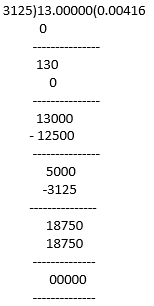13/3125 = 0.00416

(ii) 17/8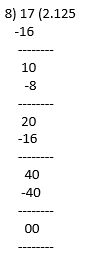17/8 = 2.125

(iii) 64/455 has a Non terminating decimal expansion

(iv)15/ 1600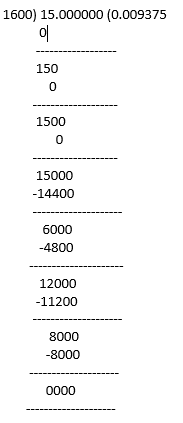15/1600 = 0.009375

(v) 29/ 343 has a Non terminating decimal expansion

(vi)23/ (2352) = 23/(8×25)= 23/200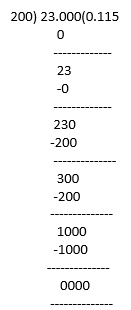23/ (2352) = 0.115

(vii) 129/ (225775) has a Non terminating decimal expansion

(viii) 6/15 = 2/5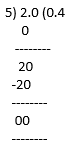(ix) 35/50 = 7/10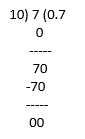35/50 = 0.7

(x) 77/210 has a non-terminating decimal expansion.

3. The following real numbers have decimal expansions as given below. In each case, decide whether they are rational or not. If they are rational, and of the form, p q what can you say about the prime factors of q?

(i) 43.123456789

(ii) 0.120120012000120000. . .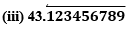Solutions:

(i) 43.123456789

Since it has a terminating decimal expansion, it is a rational number in the form of p/q and q has factors of 2 and 5 only.

(ii) 0.120120012000120000. . .

Since, it has non-terminating and non- repeating decimal expansion, it is an irrational number.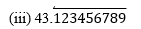Since it has non-terminating but repeating decimal expansion, it is a rational number in the form of p/q and q has factors other than 2 and 5.